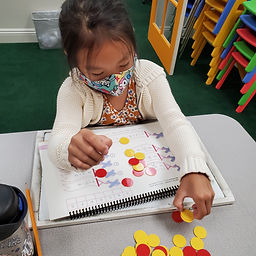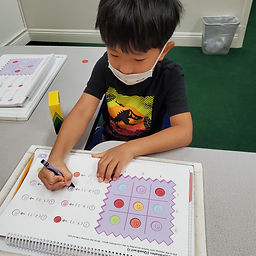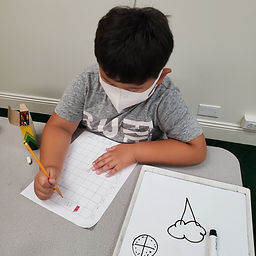## Ms. Raejean

### Target 1​

###### Lesson Type:

New

Algebra

:

Variable

Solve addition and subtraction problems where a symbol is used to represent an unknown quantity.

###### 1:

Informal introduction to variables.

###### 2:

Explain that symbols can be anything: a question mark, a blank space, a color, a shape. The symbol is just an indication of what needs to be solved.

2nd

###### Vocabulary:

Variables, Unknown

Activities:

• First students reviewed adding and subtracting numbers for fluency. Students used counters as needed.
• The term "variables" or unknown numbers was introduced. A letter or question mark represents the number we don't know. We looked at what was known and unknown in the equations.
• We talked about using subtraction to help with addition equations. 10 + ? = 17, so 17 - 10 = 7. [? = 7]
• We used counters to help us find the unknown number in a subtraction equation. 22 - ? = 20. "If you have 22 counters, but want to make 20, what would you do?" "Take away 2." [? = 2]
• Students solved problems to find the unknown.### Home Exploration

###### Guiding Questions:## Absent Students:

### Target 2

:

###### 1:

Understand that in a coordinate, the first number indicates right and left (column) placement and the second up and down (row) placement.

###### 2:

Identify a row and a column.

###### 3:

Use visual tracking to accurately find the intersection point of a row and column.

###### 4:

Explain that coordinates are used so that exact same location can be found by multiple people.

2nd

###### Vocabulary:

Coordinates, Rows, Columns

Activities:

• The concept of "coordinates" was introduced. We practiced following the row and column to find objects on a grid. We also followed the row and column to their corresponding numbers to identify the coordinates of the object.
• Students began making their own grid of objects for more practice with finding coordinates.### Home Exploration

###### Guiding Questions:### Target 3

:

###### Vocabulary:

Activities:### Home Exploration